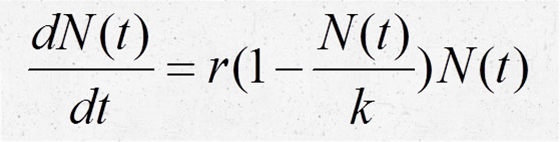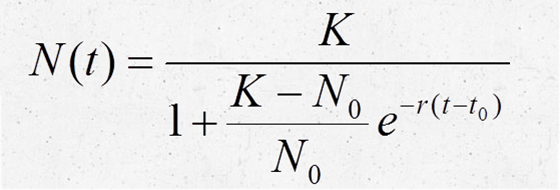# Team:HZAU-China/Modeling/Gray logistic

Gray logistic

### Aim:

To know the growth curve of Bacillus subtilis in the dog’s blood.

### Steps:

1. Experimentally measure the number of bacteria;

2. Establish the gray logistic model to simulate the growth of bacteria;

3. Determine the parameters through experiments;

4. Test the predicted results.

### Results:

The gray logistic model gives good prediction and the model precision is excellent.

### Background:

The color of blood is so deep that it is not fit to measure the OD value to determine the growth of bacteria in the blood. So we chose dilution-plate method to detect the number of total bacteria. We coated a large number of plates. If you want to know the details of the experiment, please click here. The logistic model of population can well predict the increase of population.

### Establishing the logistic model:

In the blood environment, the number of bacteria has a maximum value K. When the bacteria number approaches K, the growth rate approaches zero. Then the population growth equation is as follows:.

The solution of the equation is :,

where N0 is the size of bacterial population and r is population growth rate. For convenience, we rewrite the above equation aswhere A=K,and r is unknown parameter. N is the logarithm of the colony-forming unit (CFU) of Bacillus subtilis.

### Determining the parameters using the gray system theory:

To determine the parameters of the equation,we used the gray system theory. The equation can be rewritten as:,,;

Using the matrix equation in linear algebra we could determine the parameters α and β .

From the results, we know the value of posterior-variance is 0.1931, lower than 0.35, so that the model precision is excellent.

In conclution, the growth curve of our engineered bacterium in dog's blood is given bywhere N(t) is the logarithm of the CFU of Bacillus subtilis.

### Reference:

1.Shiqiang Zhang, China's Population Growth Model Based on Grey System Theory and Logisitic Model[C]. 2010:4. (In Chinese)

2.Xiaoyin Wang, Baoping Zhou 2010. Mathematical modeling and mathematical experiment. Beijing : Science press. (In Chinese)